### This Course has been revised!

For a more enjoyable learning experience, we recommend that you study the mobile-friendly republished version of this course.

Take me to revised course.

- or -

Continue studying this course

# Energy Systems

• Study Notes
• Discuss This Topic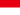Christian Gordon R. 0 0 I think the true answer is 2.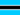Phomolo P. 0 0 When energy is needed, ATP is broken down using an enzyme (known as ATPase) into ADP (Adenosine Diphosphate). This process breaks the high energy Phosphate (P) bond and so provides energy for use by the body. Here is the equation: ATP = ADP + P +Energy The human body is also capable of resynthesising ATP to allow it to continue producing energy. To do this it must use energy to reverse the equation shown above. This is known as an endothermic reaction as it requires energy. The breakdown of ATP is called exothermic as it produces energy. The process of breaking down and resynthesising ATP is efficient at producing energy as less energy is required to resynthesis the ATP than is made to break it down. Here is the Resynthesis equation: Energy + ADP +P = ATP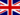Clara B. 0 0 We're is the graph to look at and compare?Clara B. 0 0 Energy systems graph?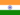Rk S. 0 0 The three energy systems:- These are the percentage contributions if you were to run as fast as possible for 10 seconds. Similarly, if you draw a line at 120 seconds, you can read off the relevant percentage contributions for this example (ATP-PC = 10%, LA = 40% and aerobic = 50%). These figures refer to the total energy contribution if you were to run as fast as possible for 120 seconds. A mistake that students often make is to analyse an event that goes for 120 seconds, read off the data and say: These percentage contribution figures are overall figures. You can only use these figures to say: "Throughout the 120 second run, the overall contribution by the energy systems was ATP-PC = 10%, LA = 40% and aerobic = 50%." Here is a question from the 1997 BOS paper for you to try. It relates to the graph above. Which of the following is true? The graph above shows that: ATP production for the first 10 seconds of a 60 second sprint will be derived almost exclusively (about 95%) from the phosphagen system. Only about 30% of energy production comes from the aerobic energy system during the first 60 seconds of a marathon. The lactacid energy system contributes approximately 50% of the total requirements for a sprint event lasting 60 seconds. All of the above are correct.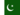Muhammad S. 0 0 What is the role of energy systems in different activities?
• Text Version